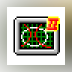•C2-Mathematics - LCM and GCD Mathematical calculator for obtaining the LCM (Least Common Multiple) and GCD... DOWNLOAD
•NH Mathematical Tools NH Mathematical Tools is a tools that help people in solving Mathematical... DOWNLOAD
•Absorb Mathematics (Viewer) Absorb Mathematics is an interactive course written by Kadie Armstrong, a... DOWNLOAD
•Basic Mathematics Basic Mathematics is an application designed to help students practice and... DOWNLOAD
•GDF Plotter - Mathematical grapher GDF is a freeware program that allows you to draw any single variable... DOWNLOAD
Apps for Mac